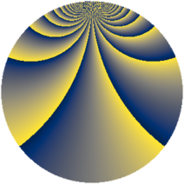# Properties

 Label 2850.2.blLevel $2850$ Weight $2$ Character orbit 2850.bl Rep. character $\chi_{2850}(199,\cdot)$ Character field $\Q(\zeta_{18})$ Dimension $360$ Sturm bound $1200$

# Learn more about

## Defining parameters

 Level: $$N$$ $$=$$ $$2850 = 2 \cdot 3 \cdot 5^{2} \cdot 19$$ Weight: $$k$$ $$=$$ $$2$$ Character orbit: $$[\chi]$$ $$=$$ 2850.bl (of order $$18$$ and degree $$6$$) Character conductor: $$\operatorname{cond}(\chi)$$ $$=$$ $$95$$ Character field: $$\Q(\zeta_{18})$$ Sturm bound: $$1200$$

## Dimensions

The following table gives the dimensions of various subspaces of $$M_{2}(2850, [\chi])$$.

Total New Old
Modular forms 3744 360 3384
Cusp forms 3456 360 3096
Eisenstein series 288 0 288

## Trace form

 $$360q + O(q^{10})$$ $$360q + 24q^{11} - 48q^{14} + 24q^{19} - 24q^{21} + 24q^{26} - 48q^{29} - 24q^{41} + 24q^{44} + 24q^{46} + 156q^{49} - 48q^{59} - 84q^{61} + 180q^{64} - 48q^{69} + 144q^{71} + 72q^{74} - 24q^{76} + 168q^{79} - 24q^{84} + 96q^{86} + 96q^{89} + 156q^{91} + 240q^{94} + 24q^{99} + O(q^{100})$$

## Decomposition of $$S_{2}^{\mathrm{new}}(2850, [\chi])$$ into newform subspaces

The newforms in this space have not yet been added to the LMFDB.

## Decomposition of $$S_{2}^{\mathrm{old}}(2850, [\chi])$$ into lower level spaces

$$S_{2}^{\mathrm{old}}(2850, [\chi]) \cong$$ $$S_{2}^{\mathrm{new}}(95, [\chi])$$$$^{\oplus 8}$$$$\oplus$$$$S_{2}^{\mathrm{new}}(190, [\chi])$$$$^{\oplus 4}$$$$\oplus$$$$S_{2}^{\mathrm{new}}(285, [\chi])$$$$^{\oplus 4}$$$$\oplus$$$$S_{2}^{\mathrm{new}}(475, [\chi])$$$$^{\oplus 4}$$$$\oplus$$$$S_{2}^{\mathrm{new}}(570, [\chi])$$$$^{\oplus 2}$$$$\oplus$$$$S_{2}^{\mathrm{new}}(950, [\chi])$$$$^{\oplus 2}$$$$\oplus$$$$S_{2}^{\mathrm{new}}(1425, [\chi])$$$$^{\oplus 2}$$